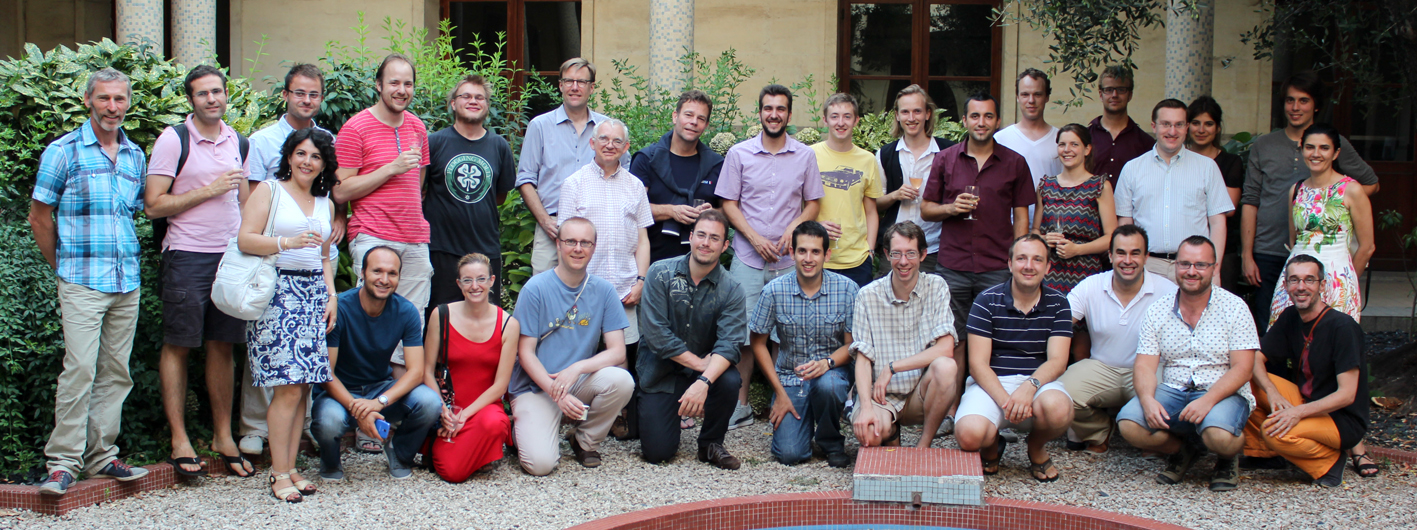# 6th SIPTA Summer SchoolMontpellier, France, 21-25 July 2014

Imprecise probability is used as a generic term to cover all mathematical or statistical models which measure chance or uncertainty without sharp numerical probabilities. It includes both qualitative models (comparative probability, partial preference orderings, fuzzy measures, ...) and quantitative models (interval-valued probabilities, convex sets of probability measures, upper and lower previsions, belief functions, possibility and plausibility measures, ...). Imprecise probability models are needed in inference problems where the relevant information is scarce, vague or conflicting, and in decision problems where preferences may also be incomplete.

The SIPTA school provides an introduction to imprecise probabilities for students and researchers. The basics and some advanced theoretical aspects will be covered. Of particular interest will be applications of imprecise probabilities. The School is organised over a one-week period in a friendly environment favouring interaction between participants. World experts in the different aspects of imprecise probabilities will teach about their area of expertise. An important part of the time is devoted to solving exercises and to the presentation of open application problems.

SIPTA (Society for Imprecise Probability: Theories and Applications) aims at promoting research on imprecise probability. This is done through a series of activities including the ISIPTA conferences every odd year since 1999, and the SIPTA schools every even year since 2004.Webmaster: Kevin Loquin <kevin.loquin@gmail.com> Last Update: November 07th, 2013